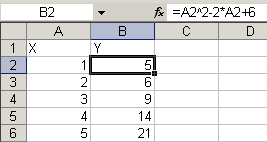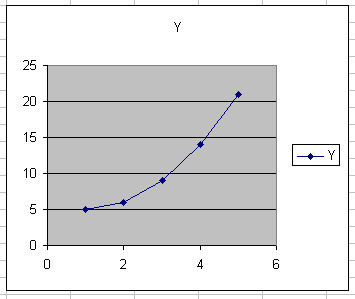## Subscribe to our mailing list

* indicates required

Audit !!!

Check out our RefTreeAnalyser
the ultimate Excel formula auditing tool.

Trainings

Excel VBA Masterclass (English)
Excel VBA for Financials (Dutch)

Third party tools

FastExcel
The best tool to optimise your Excel model!

What has changed?

This Excel compare tool is fast, reliable, and easy to use. Compare two Excel files now and see for yourself!
Home > English site > Articles > Chart an Equation > Introduction# Charting a mathematical equation using Excel and defined names

## Introduction

When doing mathematics, wouldn't it be nice if we could type an equation into a cell in Excel and immediately see the resulting graph?

Suppose we have an equation like:

y=x2-2.x+6

Normally, we would create a column with x values and in the adjacent column "translate" this formula into a cell formula:

=A2^2-2*A2+6

and copy this formula down to match the number of x values in column A:Subsequently, we would base a chart on this set of data:This method lacks flexibility. It would be much more convenient, if we could:

• Enter the (any!) formula into a single cell
• Enter the lower and upper limit of the x-range into two other cells
• Enter the number of values to use for the chart in yet another cell:Yet another constraint we're going to place on this task:

Apart from the cells shown in the figure above, no cells are to be used, just defined names with formulas.